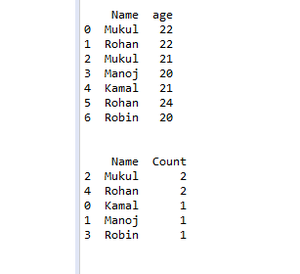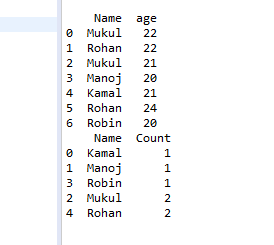Skip to content
Related Articles
Sort Dataframe according to row frequency in Pandas
• Last Updated : 24 Feb, 2021

In this article, we will discuss how to use count() and sort_values() in pandas. So the count in pandas counts the frequency of elements in the dataframe column and then sort sorts the dataframe according to element frequency.

• count(): This method will show you the number of values for each column in your DataFrame.
• sort_values(): This method helps us to sort our dataframe. In this method, we pass the column and our data frame is sorted according to this column.

Example 1: Program to sort data frame in descending order according to the element frequency.

## Python

 `# import pandas ` `import` `pandas as pd ` ` `  `# create dataframe ` `df ``=` `pd.DataFrame({``'Name'``: [``'Mukul'``, ``'Rohan'``, ``'Mukul'``, ``'Manoj'``, ` `                            ``'Kamal'``, ``'Rohan'``, ``'Robin'``], ` `                    `  `                   ``'age'``: [``22``, ``22``, ``21``, ``20``, ``21``, ``24``, ``20``]}) ` ` `  `# print dataframe ` `print``(df) ` ` `  `# use count() and sort() ` `df ``=` `df.groupby([``'Name'``])[``'age'``].count().reset_index( ` `  ``name``=``'Count'``).sort_values([``'Count'``], ascending``=``False``) ` ` `  `# print dataframe ` `print``(df) `

Output:Example 2: Program to sort data frame in ascending order according to the element frequency.

## Python

 `# import pandas ` `import` `pandas as pd ` ` `  `# create dataframe ` `df ``=` `pd.DataFrame({``'Name'``: [``'Mukul'``, ``'Rohan'``, ``'Mukul'``, ``'Manoj'``, ` `                            ``'Kamal'``, ``'Rohan'``, ``'Robin'``], ` `                    `  `                   ``'age'``: [``22``, ``22``, ``21``, ``20``, ``21``, ``24``, ``20``]}) ` ` `  `# print dataframe ` `print``(df) ` ` `  `# use count() and sort() ` `df ``=` `df.groupby([``'Name'``])[``'age'``].count().reset_index( ` `  ``name``=``'Count'``).sort_values([``'Count'``], ascending``=``True``) ` ` `  `# print dataframe ` `print``(df) `

Output:Attention geek! Strengthen your foundations with the Python Programming Foundation Course and learn the basics.

To begin with, your interview preparations Enhance your Data Structures concepts with the Python DS Course.

My Personal Notes arrow_drop_up
Recommended Articles
Page :